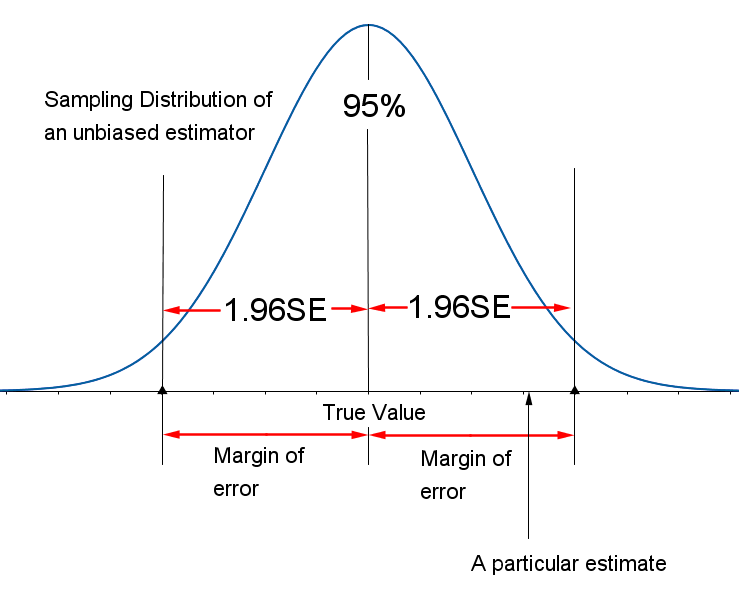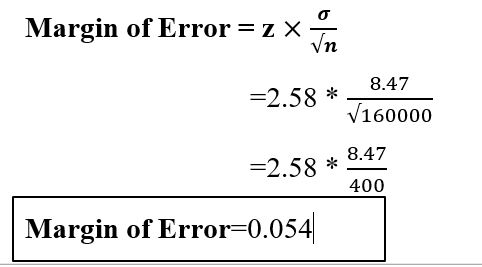# Margin Of Error###What is Margin of Error?

The Margin of error is a range of value below and above the sample statistic in a confidence interval.###Why to use Margin of Error?

Whenever we use a representative sample to make a guess something about a full population, our guess would contain some kind of uncertainty.  Using our sample statistic, we have to conclude the real statistic—and that inference will mean our guess will usually be somewhere near the actual figure (a bit too low or a bit too high, Statistics Solutions).

###How to use Margin of Error?

Formula: Margin of Error = z

Where n= sample size

σ= Population Standard Deviation

z= z-score

Example:

Find Margin of Error where sample size = 160000, Population Standard Deviation= 8.47 and z-score= 2.58The post Margin Of Error appeared first on Prwatech.

This post first appeared on Learn Big Data Hadoop In Bangalore, please read the originial post: here